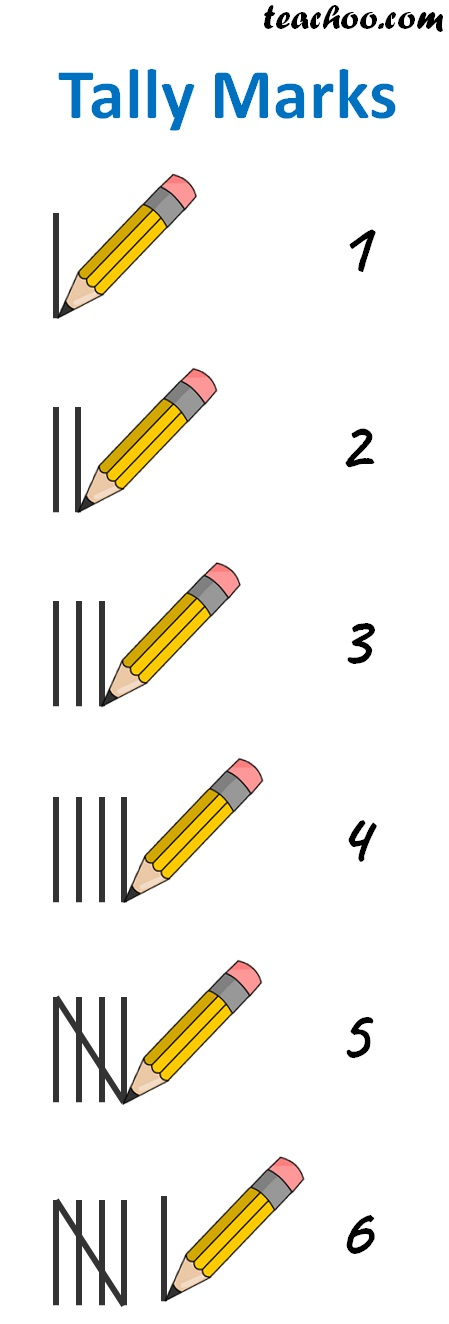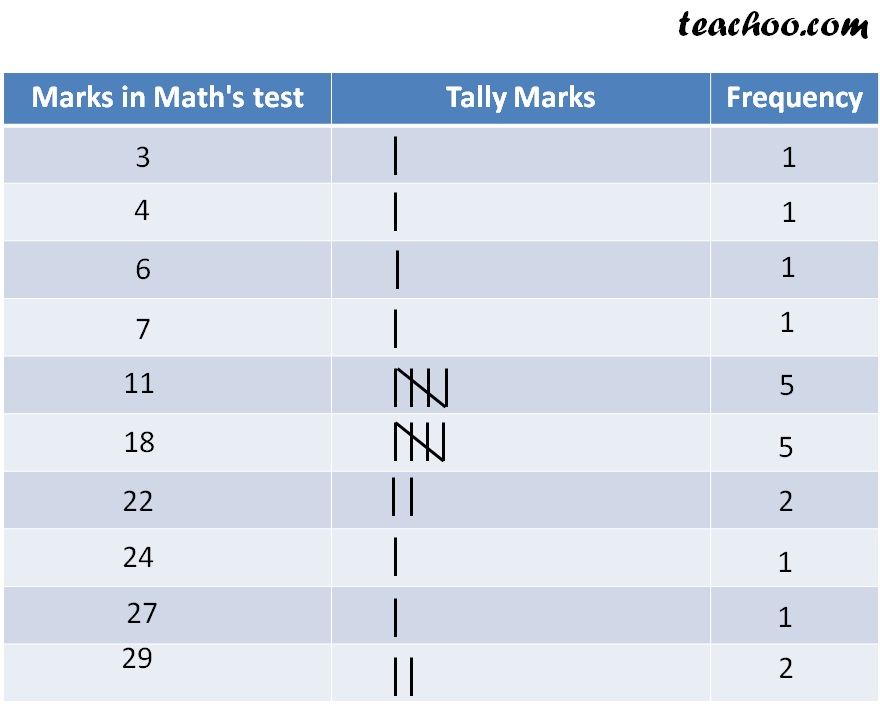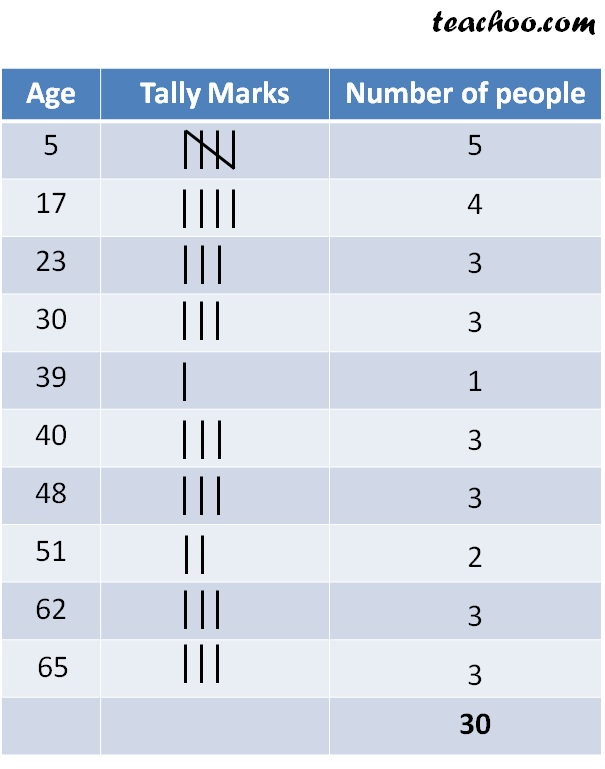Let's learn how to write Tally marks for some data.

Here is the rule we follow

• We write 1 as one line, 2 as 2 lines, 3 as 3 lines, 4 as 4 lines
• For 5, we make a bunch. So that it is easier to remember
• For 6, it is a bunch and 1 line

The Tally marks for numbers 1 to 6 is given below## Let's solve an Example

Suppose

Marks of 20 students in Maths Test

11, 4, 27, 18, 18, 3, 24, 22, 11, 22,

18, 11, 18, 7, 29, 18, 11, 6, 29, 11

We want to find number of students who scored 29? or 11?

We write the numbers in a tableSo,

Number of students who scored 29 = 2

Number of students who scored 11 = 5

## Let’s take another example

Let, following be the data of ages of 30 people in your locality

5, 65, 62, 48, 5, 23, 17, 40, 30, 30, 30, 51, 5, 17, 17, 39, 23, 48, 40, 65, 65, 62, 5, 5, 17, 62, 51, 23, 48, 40

Now organising the data using Tally marksSubscribe to our Youtube Channel - https://you.tube/teachoo

1. Chapter 5 Class 8 Data Handling
2. Concept wise
3. Frequency Distribution of Data

Transcript

We follow these steps Writing all marks in 1 column Writing 1 number as Writing 5 as

Frequency Distribution of Data